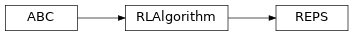# garage.tf.algos.reps¶

Relative Entropy Policy Search implementation in Tensorflow.

class REPS(env_spec, policy, baseline, discount=0.99, gae_lambda=1, center_adv=True, positive_adv=False, fixed_horizon=False, epsilon=0.5, l2_reg_dual=0.0, l2_reg_loss=0.0, optimizer=LBFGSOptimizer, optimizer_args=None, dual_optimizer=scipy.optimize.fmin_l_bfgs_b, dual_optimizer_args=None, name='REPS')Relative Entropy Policy Search.

References

 J. Peters, K. Mulling, and Y. Altun, “Relative Entropy Policy Search,”

Artif. Intell., pp. 1607-1612, 2008.

Example

\$ python garage/examples/tf/reps_gym_cartpole.py

Parameters
• env_spec (EnvSpec) – Environment specification.

• policy (garage.tf.policies.StochasticPolicy) – Policy.

• baseline (garage.tf.baselines.Baseline) – The baseline.

• scope (str) – Scope for identifying the algorithm. Must be specified if running multiple algorithms simultaneously, each using different environments and policies.

• discount (float) – Discount.

• gae_lambda (float) – Lambda used for generalized advantage estimation.

• center_adv (bool) – Whether to rescale the advantages so that they have mean 0 and standard deviation 1.

• positive_adv (bool) – Whether to shift the advantages so that they are always positive. When used in conjunction with center_adv the advantages will be standardized before shifting.

• fixed_horizon (bool) – Whether to fix horizon.

• epsilon (float) – Dual func parameter.

• l2_reg_dual (float) – Coefficient for dual func l2 regularization.

• l2_reg_loss (float) – Coefficient for policy loss l2 regularization.

• optimizer (object) – The optimizer of the algorithm. Should be the optimizers in garage.tf.optimizers.

• optimizer_args (dict) – Arguments of the optimizer.

• dual_optimizer (object) – Dual func optimizer.

• dual_optimizer_args (dict) – Arguments of the dual optimizer.

• name (str) – Name of the algorithm.

train(self, trainer)

Obtain samplers and start actual training for each epoch.

Parameters

trainer (Trainer) – Experiment trainer, which provides services such as snapshotting and sampler control.

Returns

The average return in last epoch cycle.

Return type

float